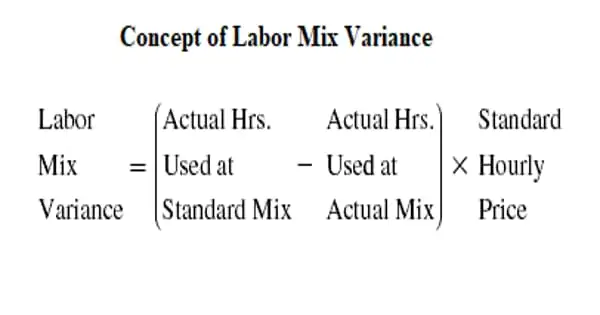Accounting

# Concept of Labor Mix VarianceThe analysis of mix variances is an essential component of cost accounting. Any significant case study or project will necessitate extensive calculations based on these variance figures. Labor mix variance, like material mix variance, is a component of labor efficiency variance. The term “labor mix,” also known as “gang,” refers to the composition of more than one grade of workers/employees. Labor mix variance, like material mix variance, is a component of labor efficiency variance.

The composition of this composition may differ between the standard and the actual. The company’s cost accountant must create a standard quantity of all these types of laborers, but the actual quantity may also be dependent on worker supply. As a result, the labor mix or gang composition variance occurs as a result of the discrepancy between the standard labor grades specified and the actual labor grades used.

Only when two or more different types of workers are employed and the composition of actual grade workers differs from the standard composition of workers does labor mix variance occur. The shift in labor composition could be due to a labor shortage in one grade. This variance indicates how much of a difference in labor costs exists as a result of the change in labor composition.

Formula for the Calculation of Labor Mix Variance (LMV)

LMV = SR x (RST – AT)

Where,

• LMV = Labor mix variance
• SR = Standard Rate
• RST = Revised standard time
• AT = Actual time

NOTE:

RST = (Total time of actual mix/Total time of standard mix) x Standard time of each grade of employees.

If the resulting figure is positive, it is referred to as favorable variance; if it is negative, it is referred to as unfavorable variance. In the event of a change in labor composition, such as a shortage or a lack of planning, labor mix variance is used to assess the impact of the change on overall workforce efficiency. As mentioned in our Labor Mix Variance or Gang Composition Variance assignment help, it is also used to analyze the overall cost variance for labor.

Only when two or more different types of workers are employed and the composition of actual grade workers differs from the standard composition of workers does labor mix variance occur. The shift in labor composition could be due to a labor shortage in one grade. Assume we set a standard of 40 skilled workers, but the actual supply was 30 of that type of worker, so we had to appoint semi-skilled workers. As a result, there will be a shift in the labor mix.